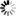Welcome to Central Library, SUST
Normal view

# Polyhedral and Algebraic Methods in Computational Geometry [electronic resource] / by Michael Joswig, Thorsten Theobald.

Material type:TextSeries: UniversitextPublisher: London : Springer London : Imprint: Springer, 2013Description: X, 250 p. 67 illus., 17 illus. in color. online resourceContent type:
• text
Media type:
• computer
Carrier type:
• online resource
ISBN:
• 9781447148173
Additional physical formats: Printed edition:: No titleDDC classification:
• 516 23
LOC classification:
• QA440-699
Online resources:
Contents:
Introduction and Overview -- Geometric Fundamentals -- Polytopes and Polyhedra -- Linear Programming -- Computation of Convex Hulls -- Voronoi Diagrams -- Delone Triangulations -- Algebraic and Geometric Foundations -- Gröbner Bases and Buchberger’s Algorithm -- Solving Systems of Polynomial Equations Using Gröbner Bases -- Reconstruction of Curves -- Plücker Coordinates and Lines in Space -- Applications of Non-Linear Computational Geometry -- Algebraic Structures -- Separation Theorems -- Algorithms and Complexity -- Software -- Notation.
Summary: Polyhedral and Algebraic Methods in Computational Geometry provides a thorough introduction into algorithmic geometry and its applications. It presents its primary topics from the viewpoints of discrete, convex and elementary algebraic geometry.   The first part of the book studies classical problems and techniques that refer to polyhedral structures. The authors include a study on algorithms for computing convex hulls as well as the construction of Voronoi diagrams and Delone triangulations.   The second part of the book develops the primary concepts of (non-linear) computational algebraic geometry. Here, the book looks at Gröbner bases and solving systems of polynomial equations. The theory is illustrated by applications in computer graphics, curve reconstruction and robotics.   Throughout the book, interconnections between computational geometry and other disciplines (such as algebraic geometry, optimization and numerical mathematics) are established.  Polyhedral and Algebraic Methods in Computational Geometry is directed towards advanced undergraduates in mathematics and computer science, as well as towards engineering students who are interested in the applications of computational geometry.
Tags from this library: No tags from this library for this title.
Star ratingsAverage rating: 0.0 (0 votes)
No physical items for this record

Introduction and Overview -- Geometric Fundamentals -- Polytopes and Polyhedra -- Linear Programming -- Computation of Convex Hulls -- Voronoi Diagrams -- Delone Triangulations -- Algebraic and Geometric Foundations -- Gröbner Bases and Buchberger’s Algorithm -- Solving Systems of Polynomial Equations Using Gröbner Bases -- Reconstruction of Curves -- Plücker Coordinates and Lines in Space -- Applications of Non-Linear Computational Geometry -- Algebraic Structures -- Separation Theorems -- Algorithms and Complexity -- Software -- Notation.

Polyhedral and Algebraic Methods in Computational Geometry provides a thorough introduction into algorithmic geometry and its applications. It presents its primary topics from the viewpoints of discrete, convex and elementary algebraic geometry.   The first part of the book studies classical problems and techniques that refer to polyhedral structures. The authors include a study on algorithms for computing convex hulls as well as the construction of Voronoi diagrams and Delone triangulations.   The second part of the book develops the primary concepts of (non-linear) computational algebraic geometry. Here, the book looks at Gröbner bases and solving systems of polynomial equations. The theory is illustrated by applications in computer graphics, curve reconstruction and robotics.   Throughout the book, interconnections between computational geometry and other disciplines (such as algebraic geometry, optimization and numerical mathematics) are established.  Polyhedral and Algebraic Methods in Computational Geometry is directed towards advanced undergraduates in mathematics and computer science, as well as towards engineering students who are interested in the applications of computational geometry.

There are no comments on this title.